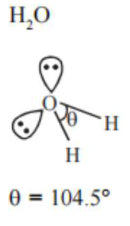# Given below are two statements: one is labelled as Assertion A and the other is labelled as

Question:

Given below are two statements: one is labelled as Assertion A and the other is labelled as

Reason $\mathrm{R}$ :

Assertion $\mathbf{A}$ : The $\mathrm{H}-\mathrm{O}-\mathrm{H}$ bond angle in water molecule is $104.5^{\circ}$

Reason $\mathbf{R}$ : The lone pair - lone pair repulsion of electrons is higher than the bond pair - bond pair repulsion.

1. $A$ is false but $R$ is true

2. Both $\mathrm{A}$ and $\mathrm{R}$ are true, but $\mathrm{R}$ is not the correct correct explanation of $\mathrm{A}$

3. A is true but $R$ is false

4. Both $A$ and $R$ are true, and $R$ is the correct explanation of $A$

Correct Option: , 4

Solution:the hybridisation of oxygen is water molecule is $\mathrm{sp}^{3}$.

So electron geometry of water molecule is tetrahedral and the bond angle should be $109^{\circ} 28^{\prime \prime}$ but as we know that lone pair-lone pair repulsion of electrons is higher than the bond pair-bond pair repulsion because lone pair is occupied more space areound central atom than that of bond pair.